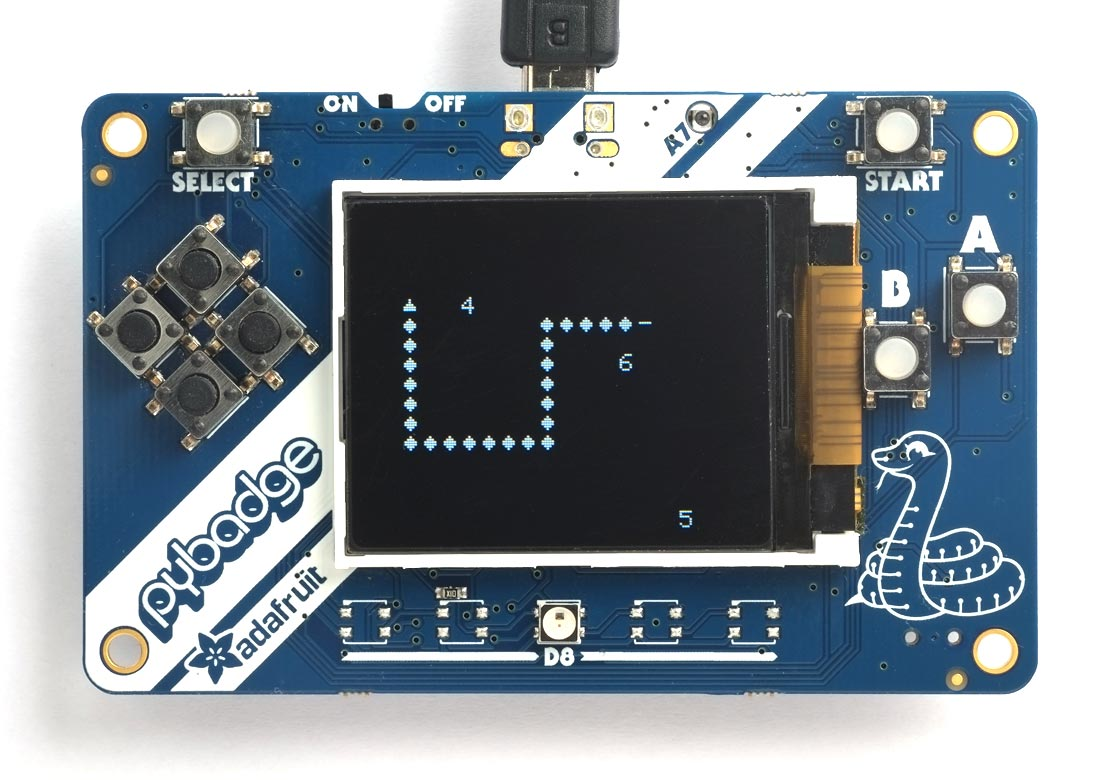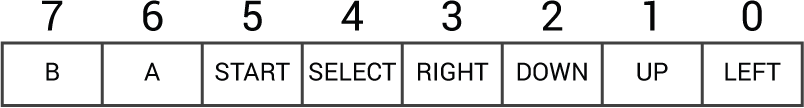This article describes a simple arcade-style game written in uLisp. The game I've chosen is a classic computer game called Snake in which you control a snake that glides around on the screen:From time to time items of food appear on the screen, and you can eat these by bumping into them, to grow the length of your snake. The aim of the game is to grow your snake as large as possible without colliding with your body.

I hope this game might inspire someone to learn Lisp, or to design their own game using this as a starting point for something more ambitious.

### Running the snake game

To run the game you need an Adafruit PyBadge LC  which is a microcontroller board based on the ATSAMD51 with GameBoy-style control buttons, and a 1.8" 160x128 colour TFT display. The game will also work on the PyBadge , or the Adafruit PyGamer which has a joystick instead of buttons .

• Include the graphics extensions before installing it by enabling the option:
`#define gfxsupport`

Alternatively here's a version that works with the PyGamer's joystick: PyGamer Snake Game.

• Select the text of the maze game, copy it, paste it into the Arduino IDE Serial Monitor input field, and press Return.
• Run the game by typing the following command into the Arduino IDE Serial Monitor input field, followed by Return:
`(play)`

Grow your snake by eating the digits, which represent food. If you bump into your body you die, and the game displays GAME OVER. Press the START button to restart a new game.

### How it works

I've intentionally kept the game as basic as possible to make it as easy as possible to understand. My original aim was to have the entire game listing fit on one side of an A4 sheet of paper, and I've just about achieved that.

The main limitations are:

• The game doesn't make use of colour. This would be a simple restriction to remedy.
• In some classic versions of the game the food disappears if you don't get to it in time. This would be easy to add.
• The game doesn't show the score, or highest score so far. This could easily be added by reducing the size of the grid by one row, and plotting this information on the top line of the display.

In this implementation of the game the display is divided into a grid of 20 x 16 cells, each of which is 8x8 pixels, and each segment of the snake is drawn in a cell. To implement the game we need to keep track of the coordinates of the head and tail of the snake, so we can move them each time the snake moves. Although the whole snake will appear to glide along, we don't actually need to move the body; we just add a body segment to the front and remove a body segment from the tail each time it moves.

We also need to keep track of the coordinates of the snake's body so we can test whether the head has collided with one of the body segments. There are two possible ways to do this. One is to keep a list of the coordinates of the snake's body segments, and check them each time the head moves. The other is to record the position of each body segment by storing a specific number in a 20 x 16 array to indicate its position on the grid. I chose the second approach as I felt it would be more efficient, since we only have to make one check to see if a collision has occurred.

Each cell on the grid contains a number to indicate what object is at that position on the grid. An empty cell contains zero. The segments of the snake contain a number between 1 and 4 to indicate which direction that segment is facing. And items of food are represented by a number between 11 and 19, to indicate an item of food with a value of between 1 and 9 respectively.

### The program

Here's an explanation of each section of the program. A full copy of the program is given at the end of the article.

#### The coordinate system

The position of each object on the 20 x 16 grid is given by its coordinates, represented as a list of two integers.

The function move returns the coordinates of a move in each of the four possible directions, N, E, S, or W, represented as an integer from 1 to 4:

`(defun move (dir) (nth dir '((0 0) (0 -1) (1 0) (0 1) (-1 0))))`

```(defun add (c1 c2)
(list
(mod (+ (first c1) (first c2)) 20)
(mod (+ (second c1) (second c2)) 16)))```

For example:

```> (add '(11 8) '(-1 0))
(10 8)```

The results are taken mod 20 and 16 respectively, to make the grid wrap round at the edges of the display.

#### Plotting the sprites

The function sprite plots an object on the display. The segments of the snake and items of food are represented on the display by plotting a character char at the appropriate grid coordinates given by coord. In addition, it stores the value cell in the appropriate element of the grid array:

```(defun sprite (grid coord cell char)
(let ((x (first coord))
(y (second coord)))
(draw-char (* 8 x) (* 8 y) char)
(setf (aref grid x y) cell)))```

The functions headsprite and tailsprite give the characters used to plot the head and tail of the snake in the four possible orientations:

```(defun headsprite (n) (nth (1- n) '(#\^ #\> #\V #\<)))
(defvar bodysprite #\O)
(defun tailsprite (n) (nth (1- n) '(#\| #\- #\| #\-)))```

An alternative option is to use graphics characters for the head and body from the built-in character set: see Graphics extensions - Character set:

```(defun headsprite (n) (nth (1- n) '(#\030 #\016 #\031 #\017)))
(defvar bodysprite #\004)
(defun tailsprite (n) (nth (1- n) '(#\| #\- #\| #\-)))```

These use the reader macro #\xxx where xxx gives the decimal value of the character.

The following routine reads the PyBadge buttons and returns a value indicating which buttons are pressed:

```(defun readbadgebuttons ()
(let ((buttons 0) (clock 48) (data 49) (latch 50))
(pinmode clock t) (digitalwrite clock 0)
(pinmode latch t) (digitalwrite latch 1)
(pinmode data nil)
(digitalwrite latch 0) (digitalwrite latch 1)
(dotimes (b 8 buttons)
(setq
buttons
(logior (ash buttons 1) (if (digitalread data) 1 0)))
(digitalwrite clock 1) (digitalwrite clock 0))))```

Each bit in the result corresponds to one of the eight possible buttons:#### Sound effects

The PyBadge LC includes an audio amplifier connected to analogue output A0, which corresponds to Arduino pin 14. By default the board includes a mini-speaker, but you can optionally disconnect this and fit a better quality speaker to the output of the amplifier.

To make sounds you first need to enable the amplifier with:

`(digitalwrite 51 1)`

The following function sound makes some simple sound effects:

```(defun sound (fy)
(dotimes (y 96) (dotimes (x (funcall fy y)) (analogwrite 14 (* 16 x)))))```

The parameter fy is a function of y that determines the type of sound. The following options give a low buzz, a rising tone, and a beep respectively:

```(defun crash (y) (* y 4))
(defun gobble (y) (- 96 y))
(defun drop (y) 32)```

#### Dropping food

The function drop-food looks for an empty cell on a random position on the grid, plots a digit from 1 to 9 there, and makes the drop sound:

```(defun drop-food (grid)
(loop
(let ((cell (list (random 20) (random 16)))
(meal (1+ (random 9))))
; Find an empty space to drop it
(when (zerop (aref grid (first cell) (second cell)))
(sprite grid cell (+ 10 meal) (code-char (+ (char-code #\0) meal)))
(sound drop)
(return))))))```

#### Game over

Finally, the function game-over prints the text "GAME OVER" in large characters in the centre of the screen:

```(defun game-over ()
(set-text-size 4)
(set-cursor 36 32) (with-gfx (str) (princ "GAME" str))
(set-cursor 36 64) (with-gfx (str) (princ "OVER" str)))```

#### The main program

Here's the main program loop. First it enables sound, and clears the screen:

```(defun play ()
; Start new game
(loop
; Enable speaker amp
(digitalwrite 51 1)
(fill-screen)```

Then it initialises the grid array, and creates the initial snake consisting of a head facing west, two body segments, and a tail:

```   ; Draw initial snake
(let ((grid (make-array '(20 16) :initial-element 0))
(tailxy '(11 8))
(direction 4) ; West
(grow 0))
(sprite grid '(9 8) direction bodysprite)
(sprite grid '(10 8) direction bodysprite)
(sprite grid tailxy direction (tailsprite direction))
```

The coordinates of the head and tail of the snake are given by headxy and tailxy, and direction specifies the direction it's moving.

The main game loop then runs approximately ten times a second to test for collisions, move the snake, drop food, and read the keys.

```     (loop
(newhead (aref grid (first hxy) (second hxy))))
```

First it sets hxy to the coordinates that the head is about to move to, and newhead gives the contents of that cell. If the contents of the cell is greater than 10 it's food. If it's anything else non-zero then we've crashed, and so return from the loop:

```        ; Test for collision
(cond
(sound gobble))
(sound crash)
(return)))```

Then we move the head in the current direction:

```        ; Move head
(sprite grid hxy 5 (headsprite direction))

If grow is zero we also move the tail. Otherwise we leave it where it is, which has the effect of growing the snake:

```        ; Move tail unless growing
(cond
((zerop grow)
(let* ((oldtail (aref grid (first tailxy) (second tailxy)))
(sprite grid tailxy 0 #\space)
(sprite grid txy (aref grid (first txy) (second txy)) (tailsprite oldtail))
(setq tailxy txy)))
(t (decf grow)))```

On average one in 100 times around the loop we drop food at a random empty position:

```        ; Maybe drop food
(when (zerop (random 100)) (drop-food grid))```

Last, we check whether any of the direction keys have been pressed, and if so change the direction:

```        ; Read keys
(let ((r
(1 4) (2 1) (4 3) (8 2) (t 0))))
(unless (or (zerop r) (= 2 (abs (- r direction))))
(setq direction r)))
(delay 100)))```

The loop ends with a 100 millisecond delay to give the correct timing. Reduce this to speed up the snake.

After a collision the game waits for two seconds, and then displays GAME OVER:

```     (delay 2000)
(game-over)
; Wait for Start button